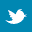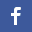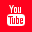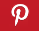Completely Solved C, C++ Programs Assignment.Quick Search Database Videos Tutorials Ebooks Forums FAQ Aboutus Household Industrial Manufacturing Service Shopping Transportation### Write a class frequency calculator to find the occurrence of the largest digit of a number within a set of numbers.

 Filed Under: C++ Assignments

PROGRAM:
Write a class frequency calculator to find the occurrence of the largest digit of a number within a set of numbers.
a) Take an array of numbers as input (dynamically allocated).
b) Then take any position of the array within the range.
c) Check whether that element exists or not, if yes then print the frequency of the largest digit within that number and also find the occurrence locations and number of occurrences.

Example:
12345 67589 92394 16547 23854
Given index=>3
The number is: 92394
The largest digit is: 9
The occurrence of the largest digit is: 2(in 1st and 4th position).

LOGICAL APPROACH:
The most important feature of c++ is the “class”. A class is an extension of the idea of structure used in c. this is a new way of creating and implementing a user-defined data type.
This c++ program is about to find the occurrence of the largest digit of a number within a set of numbers. It takes a set of numbers (using array data structure) as input. Then it takes any index of the array within the range. Here we have taken a class named “number” which includes the data member number and the member functions getdata (), maximum (). The calculation process will be in such a way that it checks whether indexed element exists or not if yes then it prints the frequency of the largest digit, the digit and the occurrence positions.
The getdata () function takes the input of the array. Maximum () function finds the largest digit, occurrence position, frequency.

ALGORITHM:
Start// algorithm to write a frequency calculator to find the occurrence of the largest digit//
Step 1: create an ADT named number. // creation of an abstract data type number//
Step 2: the data member of the ADT number is number, and the member functions are getdata() , maximum().
Step 3: defining the member functions.
getdata(i)
{
PRINT "Enter a number at index ";
INPUT number;
}
maximum()
{
max=0;
arr,i,r,c=0,count=0;
PRINT “The no is: number”;
while(number!=0)
{
r=number%10;
number=number/10;
arr[c]=r;
c++;
}
for(i=0;i<c;i++)
{
if(max<arr[i])
max=arr[i];
}
PRINT "Maximum digit is=max”;
for(i=c;i>=0;i--)
{
if(arr[i]==max)
{
PRINT (-1)*(i-c);
count++;
}
}
PRINT "Frequency of the maximum digit=count”;
}
//End of algorithm

PROGRAM LISTING:
#include<iostream>
using namespace std;
class number
{
private:
long int number;
public:
void getdata(int);
void maximum();
};
void number::getdata(int i)
{
cout<<"Enter a number at index "<<i+1<<":";
cin>>number;
}
void number::maximum()
{
long int max=0;
int arr,i,r,c=0,count=0;
cout<<"The no is:"<<number<<endl;
while(number!=0)
{
r=number%10;
number=number/10;
arr[c]=r;
c++;
}
for(i=0;i<c;i++)
{
if(max<arr[i])
max=arr[i];
}
cout<<"Maximum digit is="<<max<<endl;
cout<<”The occurrence of the maximum digit are:\n”;
for(i=c;i>=0;i--)
{
if(arr[i]==max)
{
cout<<(-1)*(i-c)<<endl;
count++;
}
}
cout<<"Frequency of the maximum digit is "<<count<<endl;

}
int main()
{
int i,j,inx;
cout<<"How many numbers want to enter?\n";
cin>>j;
number *no=new number[j];
for(i=0;i<j;i++)
no[i].getdata(i);
cout<<"\nEnter a index within the array:";
cin>>inx;
inx--;
if(inx<1 || inx>j)
cout<<"Given index is out of range...\n";
for(i=0;i<j;i++)
{
if(inx==i)
{
cout<<"\nIndex found\n";
no[i].maximum();
}
}
return 0;
}

OUTPUT:
SET 1:
How many numbers want to enter?
6
Enter a number at index 1:34565
Enter a number at index 2:292934
Enter a number at index 3:46373
Enter a number at index 4:8485687
Enter a number at index 5:45345
Enter a number at index 6:43572

Enter a index within the array:4

Index found
The no is:8485687
Maximum digit is=8
The occurrence location of the maximum digit are:
1
3
6
Frequency of the maximum digit is 3.

SET 2:
How many numbers want to enter?
5
Enter a number at index 1:456738
Enter a number at index 2:98654
Enter a number at index 3:96949591
Enter a number at index 4:3573358
Enter a number at index 5:76798

Enter a index within the array:3

Index found
The no is:96949591
Maximum digit is=9
The occurrence locations of the maximum digit are:
1
3
5
7
Frequency of the maximum digit is 4

SET 3:
How many numbers want to enter?
4
Enter a number at index 1:5675
Enter a number at index 2:67768
Enter a number at index 3:99999
Enter a number at index 4:543

Enter a index within the array:3

Index found
The no is:99999
Maximum digit is=9
The occurrence locations of the maximum digit are:
1
2
3
4
5
Frequency of the maximum digit is 5

DISCUSSIONS:
• Namespaces allow to group entities like classes, objects and functions under a name. this way the global scope can be divided into “sub-scopes”, each one with its own name.

• All files in the c++ standard library declare all of its entities within the std namespace. That is why we have generally included the using namespace std;statement in all programs that used any entity defined in iostream.

• Memory allocation done dynamically using new operator as classname*objectname=newclassname(number of objects).

• A class is an extension of the idea of structure used in C. C++ supports all the features of structures as defined in c. but c++ has expanded its capabilities further to suit its OOP philosophy. It attemps to bring the user-defined typesas close an possible to the built-in data types, and also provides a facility to hide the data which is one of the main principles of OOP.Back to main directory:Software Practical